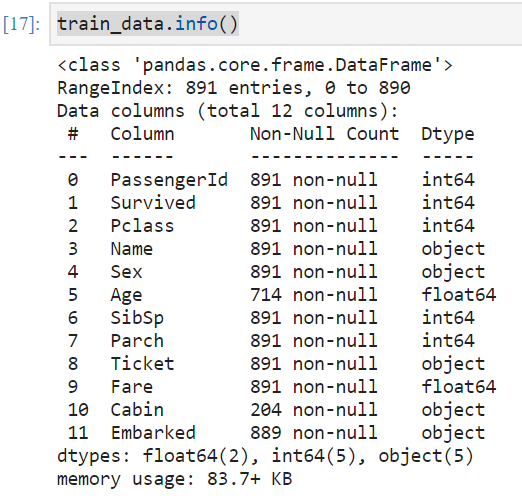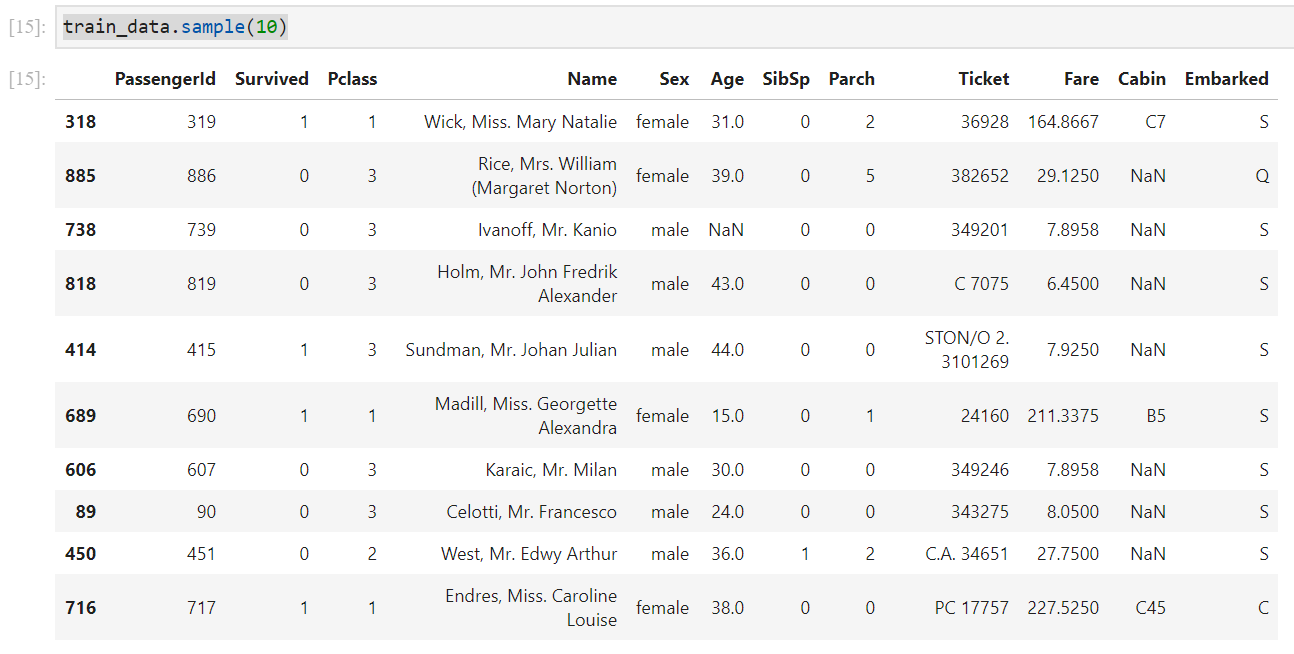signed

QiShunwang

“诚信为本、客户至上”

2020/8/19 23:52:28   来源：

## 数据加载及探索性数据分析

### 数据加载

``````pd.read_csv()：读取以‘，’分割的文件到DataFrame,用于读取csv文件(csv用逗号符分隔字符段)
``````

1. 导入numpy、pandas和matplotlib
``````import numpy as np
import pandas as pd
import matplotlib.pyplot as plt
``````
1. 载入数据
``````data_path = 'C:/Users/87569/Desktop/Titanic/'	#设置数据集路径
train_data = pd.read_csv(data_path + 'train.csv',delimiter = ',')
test_data = pd.read_csv(data_path + 'test.csv',delimiter = ',')
``````
1. 简略观察数据情况

对应的数据名称``````train_data.info()
``````info()熟悉数据类型
通过info()来了解数据每列的type，有助于了解是否存在除了nan以外的特殊符号异常。也能观察到数据的shape，例如图中可以看见数据为891行，12列。

``````train_data.sample(10)		#随机抽取10行数据展示
````````````train_data.describe()
``````describe()熟悉相关统计量
describe()中包含每列的统计量，个数(count)、平均值(mean)、方差(std)、最小值(min)、中位数(25% 50% 75%)、最大值(max)等。通过观察以上指标，可以瞬间掌握数据的大概范围和每个值的异常值的判断 。

``````chunker = pd.read_csv('train.csv', chunksize=10) 	#逐块读取
``````

1. 将表头改成中文，索引改为乘客ID

``````df = pd.read_csv('train.csv', names=['乘客ID','是否幸存','仓位等级','姓名','性别','年龄','兄弟姐妹个数','父母子女个数','船票信息','票价','客舱','登船港口'],index_col='乘客ID',header=0)
df.sample(10)
``````4.2 修改DataFrame的columns或index属性值

``````df2.columns = ['乘客ID','是否幸存','仓位等级','姓名','性别','年龄','兄弟姐妹个数','父母子女个数','船票信息','票价','客舱','登船港口']
df2.sample(5)
``````4.3 pandas.DataFrame.rename()函数
rename函数是专门为了修改DataFrame坐标轴标签函数。rename函数的优点：可以选择性的修改某行某列的标签。

函数/字典中的值必须是唯一的（1对1）。 未包含在字典/Series中的标签将保留原样。列出的额外标签不会引发错误。

``````DataFrame.rename(self, mapper=None, index=None, columns=None, axis=None, copy=True, nplace=False, level=None, errors='ignore')
``````

### 查看数据情况

1. 查看缺失值
``````missing_data = df.isnull().sum()

missing_data = missing_data[missing_data>0]

print(missing_data)

missing_data.plot.bar()
````````````isnull()   			若值为空则返回1，否则返回0
isnull().sum()		可以计算缺失值并输出缺失值个数
``````
1. 查看每种数据的差异数

``````df['仓位等级'].unique()
``````### 保存数据

``````df.to_csv('train_chinese.csv')
``````## 查看test.csv文件## 探索性数据分析EDA

EDA的目标

1. 熟悉数据集，了解数据集，对数据集进行验证来确定所获得数据集可以用于接下来的机器学习或者深度学习使用。
2. 了解变量间的相互关系以及变量与预测值之间的存在关系。
3. 引导数据科学从业者进行数据处理以及特征工程的步骤,使数据集的结构和特征集让接下来的预测问题更加可靠。

### 缺失值处理

``````年龄（age）     	 	177

``````

``````Age       86
Fare       1
Cabin    327
``````

①　年龄（Age）里面数据总数是1046条，缺失了1309-1046=263，缺失率263/1309=20%；
②　船票价格（Fare）里面数据总数是1308条，缺失了1条数据；

③　登船港口（Embarked）里面数据总数是1307，只缺失了2条数据，缺失比较少；
④　客舱（Cabin）里面数据总数是295，缺失了1309-295=1014，缺失率=1014/1309=77.5%，缺失比较大。

``````fillna(value=None, method=None, axis=None, inplace=False, limit=None, downcast=None, **kwargs)
``````
• 参数：value : 变量, 字典, Series, or DataFrame
用于填充缺失值（例如0），或者指定为每个索引（对于Series）或列（对于DataFrame）使用哪个字典/Serise/DataFrame的值。（不在字典/Series/DataFrame中的值不会被填充）这个值不能是一个列表。

• method : {‘backfill’, ‘bfill’, ‘pad’, ‘ffill’, None}, 默认值 None ; 在Series中使用方法填充空白（‘backfill’, ‘bfill’向前填充，‘pad’, ‘ffill’向后填充）

• axis : {0 or ‘index’, 1 or ‘columns’}

• inplace : boolean, 默认值 False。如果为Ture,在原地填满。注意：这将修改次对象上的任何其他视图（例如，DataFrame中的列的无复制贴片）

• limit : int, 默认值 None； 如果指定了方法，则这是连续的NaN值的前向/后向填充的最大数量。 换句话说，如果连续NaN数量超过这个数字，它将只被部分填充。 如果未指定方法，则这是沿着整个轴的最大数量，其中NaN将被填充。 如果不是无，则必须大于0。

• downcast : dict, 默认是 None； 如果可能的话，把 item->dtype 的字典将尝试向下转换为适当的相等类型的字符串（例如，如果可能的话，从float64到int64）

``````##训练集和测试集合并处理
full_data = train_data.append(test_data,ignore_index='Ture')
full_data.info()
````````````##数值型缺失值处理
full_data['Age'].fillna(midage,inplace=True)   ##通过平均值来填补缺失值

print(full_data['Age'].isnull().sum()) #0
``````

df.where(df[‘Fare’].not_eq(nil) & df[‘Pclass’].eq(3) & df[‘Cabin’].eq(nil) )[‘Fare’].median => 8.05

Cabin 缺失的比较多，但发现相同Cabin的Ticket也相同，因此可以反推具有相同Ticket的乘客也会住在同一个Cabin内，因此可以找出具有相同Ticket但Cabin不为空的乘客数据来填补：

df[‘Cabin’].missing => 1014 same_cabins = 0 df.where(df[‘Cabin’].eq(nil)).each_row_with_index do |row, idx| same_cabin = df.where( df[‘Cabin’].not_eq(nil) & df[‘Ticket’].eq(row[‘Ticket’]) ) if same_cabin.size != 0 same_cabins = 1 end end same_cabins # => 16

``````在这里插入代码片
``````